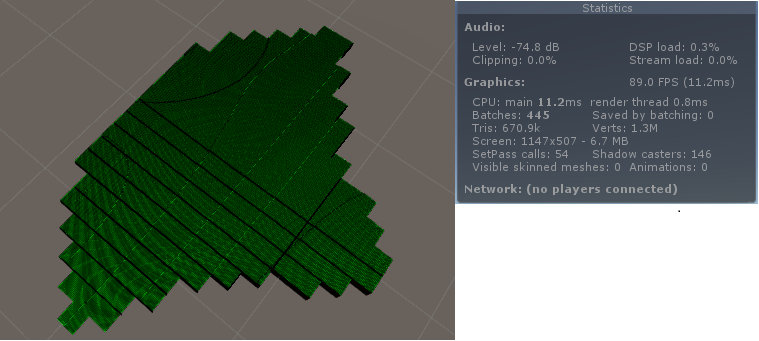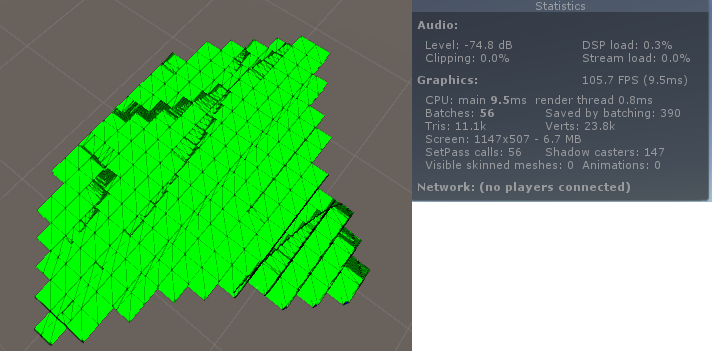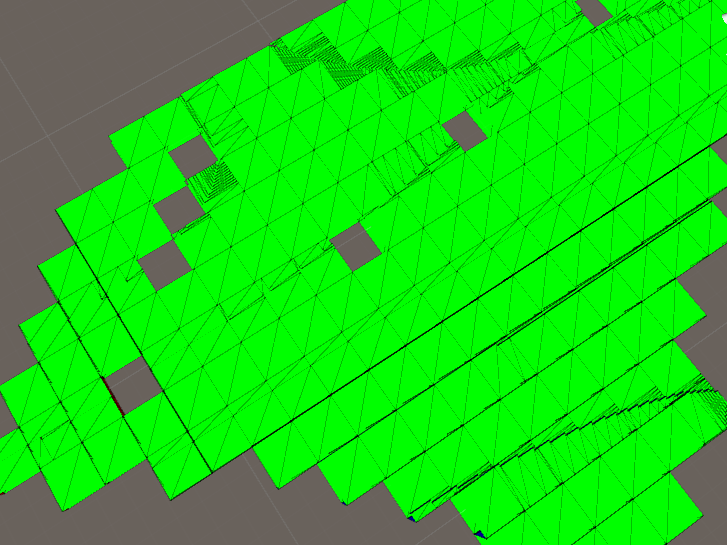# Procedural World - Mesh Optimization

## Better Naive Block Meshing

The Naive/Simple block mesher creates triangles for each block in the mesh with some minor optimization.

The simple block mesher checks if adjacent blocks to the current block are present. If they are, the connecting face is not generated because it is hidden. This is good however the algorithm does not check if there is an adjacent block in a neighboring chunk.

I provided a way for neighboring chunks to be query seemlessly from the algorithm.

public float GetField(int x, int y, int z)
{
if (x < 0)
{
return GetNeighborField(Direction.Left, x + chunkSizeX, y, z);
}
else if (x >= chunkSizeX)
{
return GetNeighborField(Direction.Right, x - chunkSizeX, y, z);
}

if (y < 0)
{
return GetNeighborField(Direction.Bottom, x, y + chunkSizeY, z);
}
else if (y >= chunkSizeY)
{
return GetNeighborField(Direction.Top, x, y - chunkSizeY, z);
}

if (z < 0)
{
return GetNeighborField(Direction.Near, x, y, z + chunkSizeZ);
}
else if (z >= chunkSizeZ)
{
return GetNeighborField(Direction.Far, x, y, z - chunkSizeZ);
}

return field.Get(x, y, z);
}

private float GetNeighborField(Direction d, int x, int y, int z)
{
var chunk = GetNeighbor(d);

if (chunk != null)
{
return chunk.GetField(x, y, z);
}
else
{
return -1;
}
}



## Greedy Meshing

The following is Mikola Lysenko’s Greedy meshing implemention ported by me to C#/Unity.

## Greedy and Naive Meshing ComparisonStat Naive Greedy
tris 670k 11.1k
verts 1.3M 23.8k

That’s a huge reduction in triangles and vertices!

I think this will simplify mesh collision a lot!Well as it turns out the mask variable in the greedy meshing algorithm can overflow. This being C# I suspect an exception is thrown but since it is running in another thread it fails silently.
Simple solution was to increase the mask size by 1 in each dimension.